NEET  >  31 Year NEET Previous Year Questions: Oscillations -2

# 31 Year NEET Previous Year Questions: Oscillations -2

Test Description

## 30 Questions MCQ Test Physics Class 11 | 31 Year NEET Previous Year Questions: Oscillations -2

31 Year NEET Previous Year Questions: Oscillations -2 for NEET 2023 is part of Physics Class 11 preparation. The 31 Year NEET Previous Year Questions: Oscillations -2 questions and answers have been prepared according to the NEET exam syllabus.The 31 Year NEET Previous Year Questions: Oscillations -2 MCQs are made for NEET 2023 Exam. Find important definitions, questions, notes, meanings, examples, exercises, MCQs and online tests for 31 Year NEET Previous Year Questions: Oscillations -2 below.
Solutions of 31 Year NEET Previous Year Questions: Oscillations -2 questions in English are available as part of our Physics Class 11 for NEET & 31 Year NEET Previous Year Questions: Oscillations -2 solutions in Hindi for Physics Class 11 course. Download more important topics, notes, lectures and mock test series for NEET Exam by signing up for free. Attempt 31 Year NEET Previous Year Questions: Oscillations -2 | 30 questions in 60 minutes | Mock test for NEET preparation | Free important questions MCQ to study Physics Class 11 for NEET Exam | Download free PDF with solutions
 1 Crore+ students have signed up on EduRev. Have you?
31 Year NEET Previous Year Questions: Oscillations -2 - Question 1

### A particle moving along the X-axis, executes simple harmonic motion then the force acting on it is given by 

Detailed Solution for 31 Year NEET Previous Year Questions: Oscillations -2 - Question 1

For simple harmonic motion, F = – kx.
Here,  k = Ak.

31 Year NEET Previous Year Questions: Oscillations -2 - Question 2

### A particle, with restoring force proportional to displacement and resistve force proportional to velocity is subjected to a force F sin ω0. If the amplitude of the particle is maximum for ω = ω1 and the energy of the particle is maximum for ω = ω2, then [1989,1998]

Detailed Solution for 31 Year NEET Previous Year Questions: Oscillations -2 - Question 2

At maximum energy of the particle, velocity resonance takes place, which occurs when frequency of external periodic force is equal to natural frequency of undamped vibrations, i.e. ω2 = ω0.
Further, amplitude resonance takes place at a frequency of external force which is less than the frequency of undamped natural vibrations, i.e. ω1 ≠  ω0 .

31 Year NEET Previous Year Questions: Oscillations -2 - Question 3

### A mass m is suspen ded from a two coupled springs, connected in series. The force constant for springs are k1 and k2. The time period of the suspended mass will be 

Detailed Solution for 31 Year NEET Previous Year Questions: Oscillations -2 - Question 3

The effective spring constant of two springs in series;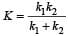Time period,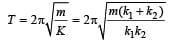31 Year NEET Previous Year Questions: Oscillations -2 - Question 4

The composition of two simple h ar monic motions of equal periods at right angle to each other and with a phase difference of π results in the displacement of the particle along 

Detailed Solution for 31 Year NEET Previous Year Questions: Oscillations -2 - Question 4

x = a sinωt and y = b sin (ωt + π) = – b sinωt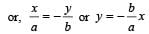It is an equatation of a straight line.

31 Year NEET Previous Year Questions: Oscillations -2 - Question 5

The angular velocity and the amplitude of a simple pendulum is ω and a respectively. At a displacement x from the mean position if its kinetic energy is T and potential energy is V, then the ratio of T to V is 

Detailed Solution for 31 Year NEET Previous Year Questions: Oscillations -2 - Question 5

PE ,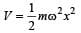and K.E.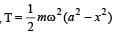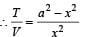31 Year NEET Previous Year Questions: Oscillations -2 - Question 6

A body is executing S .H. M . When the displacements from the mean position are 4cm and 5 cm, the corresponding velocities of the body are 10 cm per sec and 8 cm per sec. Then the time period of the body is 

Detailed Solution for 31 Year NEET Previous Year Questions: Oscillations -2 - Question 6

For S.H.M., Velocity,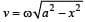at displacement x.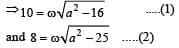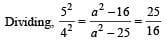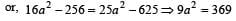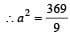Putting this value in equation (2) mentioned above,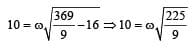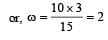radian / sec .

Time period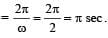31 Year NEET Previous Year Questions: Oscillations -2 - Question 7

A simple pendulum is suspended from the roof of a trolley which moves in a horizontal direction with an acceleration a, then the time period is given by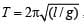, where g is equal to 

Detailed Solution for 31 Year NEET Previous Year Questions: Oscillations -2 - Question 7

The effective value of acceleration due to gravity is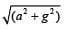31 Year NEET Previous Year Questions: Oscillations -2 - Question 8

If a simple harmonic oscillator has got a displacement of 0.02 m and acceleration equal to 2.0 m/s2 at any time, the angular frequency of the oscillator is equal to 

Detailed Solution for 31 Year NEET Previous Year Questions: Oscillations -2 - Question 8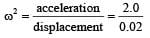ω2= 100 or ω = 10 rad/s

31 Year NEET Previous Year Questions: Oscillations -2 - Question 9

A simple harmonic oscillator has an amplitude A and time period T. The time required by it to travel from x = A to x = A/2 is 

Detailed Solution for 31 Year NEET Previous Year Questions: Oscillations -2 - Question 9

For S.H.M.,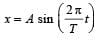When x = A,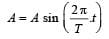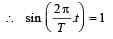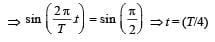When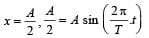or,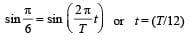Now, time taken to travel from x = A to x = A/2 is  (T/4 – T/12) = T/6

31 Year NEET Previous Year Questions: Oscillations -2 - Question 10

A wave has S.H.M (Simple Harmonic Motion) whose period is 4 seconds while another wave which also possess SHM has its period 3 seconds. If both are combined, then the resultant wave will have the period equal to 

Detailed Solution for 31 Year NEET Previous Year Questions: Oscillations -2 - Question 10

Beats are produced. Frequency of beats will be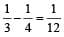Hence time period = 12 s.

[Alt : Frequency of combined S.H.M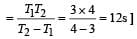31 Year NEET Previous Year Questions: Oscillations -2 - Question 11

A body executes S.H.M with an amplitude A. At what displacement from the mean position is the potential energy of the body is one fourth of its total energy ? 

Detailed Solution for 31 Year NEET Previous Year Questions: Oscillations -2 - Question 11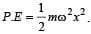Total energy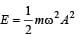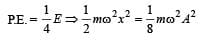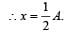[Alt : The displacement at which the P.E. becomes n times the total energy is given by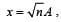where A is the amplitude.]

31 Year NEET Previous Year Questions: Oscillations -2 - Question 12

Which one of the following is a simple harmonic motion? 

Detailed Solution for 31 Year NEET Previous Year Questions: Oscillations -2 - Question 12

A wave moving through a string fixed at both ends, is a transverse wave formed as a result of simple harmonic motion of particles of the string.

31 Year NEET Previous Year Questions: Oscillations -2 - Question 13

A par ticle is subjected to two mutually perpendicular simple harmonic motions such that its x and y coordinates are given by

x = 2 sin ωt;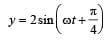The path of the particle will be 

Detailed Solution for 31 Year NEET Previous Year Questions: Oscillations -2 - Question 13

As phase difference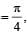the resultant path of particle is an ellipse.

31 Year NEET Previous Year Questions: Oscillations -2 - Question 14

In a simple harmonicmotion, when the displacement is one-half the amplitude, what fraction of the total energy is kinetic? 

Detailed Solution for 31 Year NEET Previous Year Questions: Oscillations -2 - Question 14

Total energy of particle executing S.H.M. of amplitude (A).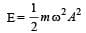K.E.of the particle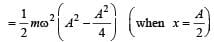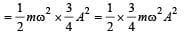Clearly ,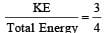[Alt : If the displacement be n times the amplitude, then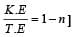31 Year NEET Previous Year Questions: Oscillations -2 - Question 15

A linear harmonic oscillator of force constant 2 × 106 N/m and amplitude 0.01 m has a total mechanical energy of 160 J. Its 

Detailed Solution for 31 Year NEET Previous Year Questions: Oscillations -2 - Question 15

k = 2 × 106 N/m
Amplitude (A) = 0.01 m
Total mechanical energy = 160J
As we know that total mechanical energy is equal to total maximum potential energy.
Maximum K. E.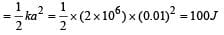31 Year NEET Previous Year Questions: Oscillations -2 - Question 16

A particle starts simple harmonic motion from the mean position. Its amplitude is A and time period is T.What is its displacement when its speed is half of its maximum speed 

Detailed Solution for 31 Year NEET Previous Year Questions: Oscillations -2 - Question 16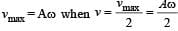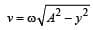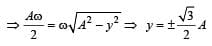[Alt : The displacement at which the speed is n times the maximum speed is given by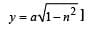31 Year NEET Previous Year Questions: Oscillations -2 - Question 17

If the length of a simple pendulum is increased by 2%, then the time period 

Detailed Solution for 31 Year NEET Previous Year Questions: Oscillations -2 - Question 17

We know that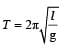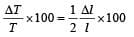If length is increased by 2%., time period increases by 1%.

31 Year NEET Previous Year Questions: Oscillations -2 - Question 18

Two  simple harmonic motions with the same frequency act on a particle at right angles i.e., along x and y axis. If the two amplitudes are equal and the phase difference is ω/2, the resultant motion will be 

Detailed Solution for 31 Year NEET Previous Year Questions: Oscillations -2 - Question 18

Equation of two simple harmonic motions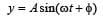....(1)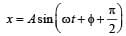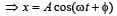....(2)

On squaring and adding equations  (1) and (2),
x2 + y2 = A2

This is an equation of a circle. Hence, resulting motion will be a circular motion.

31 Year NEET Previous Year Questions: Oscillations -2 - Question 19

A hollow sphere is filled with water. It is hung by a long thread. As the water flows out of a hole at the bottom, the period of oscillation will 

Detailed Solution for 31 Year NEET Previous Year Questions: Oscillations -2 - Question 19

Time period of simple pendulum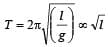where l is effective
length. [i.e distance between centre of suspension and centre of gravity of bob]

Initially, centre of gravity is at the centre of sphere. When water leaks the centre of gravity goes down until it is half filled; then it begins to go up and finally it again goes at the centre. That is effective length first increases and then decreases. As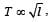, so time period first increases and then decreases.

31 Year NEET Previous Year Questions: Oscillations -2 - Question 20

Two simple pendulums of length 5m and 20m respectively are given small linear displacement in one direction at the same time. They will again be in the phase when the pendulum of shorter length has completed ....... oscillations 

Detailed Solution for 31 Year NEET Previous Year Questions: Oscillations -2 - Question 20

Let the pendulums be in phase after t sec of start. Within this time, if the bigger pendulum executes n oscillations, the smaller one will have executed (n + 1) oscillations.
Now, the time of n oscillation =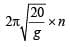& the time of (n + 1) oscillation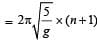To be in phase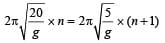or,      2n = n + 1
or,      n = 1
Hence, the no. of oscillations executed by shorter pendulum = n + 1 = 1 + 1 = 2

31 Year NEET Previous Year Questions: Oscillations -2 - Question 21

A mass m is vertically suspended from a spring of negligible mass; the system oscillates with a frequency n. What will be the frequency of the system, if a mass 4 m is suspended from the same spring? 

Detailed Solution for 31 Year NEET Previous Year Questions: Oscillations -2 - Question 21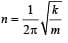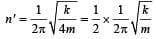On putting the value of n  we get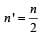31 Year NEET Previous Year Questions: Oscillations -2 - Question 22

The time period of a simple pendulum is 2 seconds. If its length is increased by 4-times, then its period becomes 

Detailed Solution for 31 Year NEET Previous Year Questions: Oscillations -2 - Question 22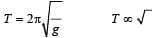If  is increased by 4 times, time period will increase by two times.

31 Year NEET Previous Year Questions: Oscillations -2 - Question 23

A particle executing S.H.M. has amplitude 0.01m and frequency 60 Hz. The maximum acceleration of the particle is 

Detailed Solution for 31 Year NEET Previous Year Questions: Oscillations -2 - Question 23

Amplitude (A) = 0.01 m, Frequency = 60 Hz
Maximum acceleration = Aω2 = 0.01 x (2πn)2
= 0.01 x 4π2 x 60 x 60 = 144π2 m/sec2

31 Year NEET Previous Year Questions: Oscillations -2 - Question 24

Masses MA and MB hanging from the ends of strings of lengths LA and LB are executing simple harmonic motions. If their frequencies are fA = 2fB, then 

Detailed Solution for 31 Year NEET Previous Year Questions: Oscillations -2 - Question 24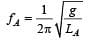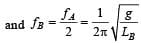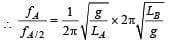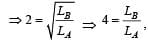regardless of mass.

31 Year NEET Previous Year Questions: Oscillations -2 - Question 25

Two simple harmonic motions act on a particle.
These harmonic motions are x = A cos (ωt + δ), ythe resulting motion is 

Detailed Solution for 31 Year NEET Previous Year Questions: Oscillations -2 - Question 25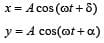....(1)

When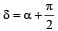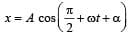x = -A sin (ωt + α)            ....(2)
Squaring (1) and (2)
and then adding x2 + y2 = A2 [cos2 (ωt +α) + sin2 (ωt +α)] or x2 + y2 = A2, which is the equation of a circle.
The present motion is anticlockwise.

31 Year NEET Previous Year Questions: Oscillations -2 - Question 26

A simple pendulum has a metal bob, which is negatively charged. If it is allowed to oscillate above a positively charged metallic plate, then its time period will 

Detailed Solution for 31 Year NEET Previous Year Questions: Oscillations -2 - Question 26

We know that the time period (T)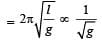Since the negatively charged bob is attracted by the positively charged plate, therefore acceleration due to gravity will increase and time period will decrease.

31 Year NEET Previous Year Questions: Oscillations -2 - Question 27

There is a body having mass m and performing S.H.M. with amplitude a. There is a restoring force F = –kx. The total energy of body depends upon

Detailed Solution for 31 Year NEET Previous Year Questions: Oscillations -2 - Question 27

Total Energy of body perfor ming simple harmonic motion =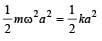where k = mω2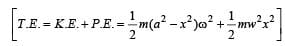Hence energy depends upon amplitude and k (spring constant).

31 Year NEET Previous Year Questions: Oscillations -2 - Question 28

A body of mass M, executes vertical SHM with periods t1 and t2, when separately attached to spring A and spring B respectively. The period of SHM, when the body executes SHM, as shown in the figure is t0. Then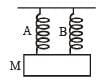Detailed Solution for 31 Year NEET Previous Year Questions: Oscillations -2 - Question 28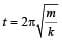⇒  k = Const.t-2

Here the springs are joined in parallel. So k0 = k1+k2 where k0 is resultant force constant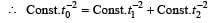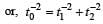31 Year NEET Previous Year Questions: Oscillations -2 - Question 29

The amplitude of a pendulum executing damped simple harmonic motion falls to 1/3 the original value after 100 oscillations. The amplitude falls to S times the original value after 200 oscillations, where S is 

Detailed Solution for 31 Year NEET Previous Year Questions: Oscillations -2 - Question 29

In damped harmonic oscillator, amplitude falls exponentially.

After 100 oscillations amplitude falls to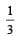times.

∴ After next 100 oscillations i.e., after 200 oscillations amplitude falls to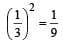times.

31 Year NEET Previous Year Questions: Oscillations -2 - Question 30

A particle is executing a simple harmonic motion of amplitude a. Its potential energy is maximum when the displacement from the position of the maximum kinetic energy is 

Detailed Solution for 31 Year NEET Previous Year Questions: Oscillations -2 - Question 30

P.E. of particle executing S.H.M. =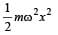At x = a, P.E. is maximum i.e.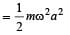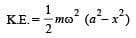At x = 0, K.E. is maximum.

Hence, displacement from position of maximum Kinetic energy = ±a.

## Physics Class 11

124 videos|464 docs|210 tests
Information about 31 Year NEET Previous Year Questions: Oscillations -2 Page
In this test you can find the Exam questions for 31 Year NEET Previous Year Questions: Oscillations -2 solved & explained in the simplest way possible. Besides giving Questions and answers for 31 Year NEET Previous Year Questions: Oscillations -2, EduRev gives you an ample number of Online tests for practice

## Physics Class 11

124 videos|464 docs|210 tests

### How to Prepare for NEET

Read our guide to prepare for NEET which is created by Toppers & the best Teachers(Scan QR code)